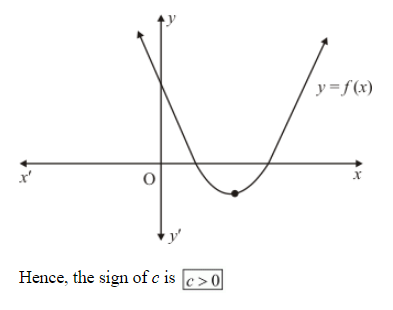# In Q. No. 14, write the sign of c.`
Question:

In Q. No. 14, write the sign of c.

Solution:

The parabola $y=a x^{2}+b x+c$ cuts $y$-axis at point P which lies on $\mathrm{y}$-axis. Putting $x=0$ in $y=a x^{2}+b x+c$, we get $y=c$. So the coordinates of P are $(0, c)$. Clearly, P lies on OY. Therefore $c>0$